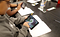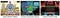# How do people use math today?Students working on the BrainQuake Gears puzzle (in the original Wuzzit Trouble app). The more advanced Gears puzzles do not have unique “right” answers. They are all about finding optimal solutions under a constraint. You can optimize the number of bonus items collected (and in some cases the number of penalty items avoided), and the number of moves taken, but you have to collect all your bonus items before you collect the final key. That requires a range of important 21st Century math skills.

I don’t know the answer to that question. Other than to say they use it in lots of different ways.

I can say that, in many cases, people use math without knowing it, since we have succeeded in creating mathematical tools that where the math is completely hidden.

Google is one example. Search engines are implementations of mathematical algorithms — more complex versions of the familiar procedures we have for adding, multiplying, subtracting, and dividing numbers. Similarly, when we look for items to buy on Amazon or any online store and then place an order, we are instructing mathematical algorithms to meet our needs. In fact, almost anything we do online involves us setting in motion a sequence of mathematical activities.

But what about uses of math where the person doing it does more than just press keys or select icons on a smart-screen? What kinds of problems do people work on where they need to have, and use, math skills?

Again, I’d have to answer that they use math in lots of different ways, on a wide range of problems. I can however be more precise in saying what I think* are the kinds of problems where people most frequently use math skills. They are, for the most part, not problems that require calculation of an answer — be that answer a number, a mathematical formula, or a graph. Rather they are optimization problems, where the goal is do something faster, cheaper, safer, more efficiently, more accurately, or better in some other way.

[* I admit I do not know for a fact that the majority of applications of math today are optimization problems. I’m not even sure it would be possible to conduct a study to find that out, since math can get into issues in many different ways and to very different extents, so it’s not clear what you should count as a math application. But I do know from my own experience working as a professional mathematician for almost half a century, that optimization problems loom very large on the spectrum of real-life applications of math, and my sense is that they are in fact the majority where the math involvement is dominant.]

The point is, the basic mathematical skillset required to work on optimization problems (faster, cheaper, safer, more efficiently, more accurately, or better in some other way) is not the one that was dominant before the world had comuters. Rather, it includes skills such as number sense, mathematical thinking, creative problem solving ability (including problems that require multiple steps to solve), a familiarity with mathematical abstractions, and a positive attitude to mathematics coupled with a sense of mathematical efficacy (mindset).

Notably, the list does not include arithmetic and algebra skills. Computers can do those today. (Though be careful. Students almost certainly need to spend some time learning how to do arithmetic and algebra, in order to acquire the skills I listed in that last paragraph. Arithmetic and algebra are not going away. But the goal of that learning is no longer because students will need to execute the various procedures mentally or by hand. The focus today has to be on understanding rather than speed, fluency, or accuracy of execution.)

Beyond that basic skills list, there are some specialized branches of mathematics that are used over-and-over again, the top of the list for optimization problems being linear algebra. But those speciality disciplines can vary from one problem domain to another.

As I have mentioned on this blog a number of times, that list of basic, 21st Century mathematical skills is precisely the ones we design our math learning tools to help develop. We founded BrainQuake precisely to provide 21st Century learning tools that could meet that 21st Century need.The three puzzles in the new BrainQuake app present challenges in positive whole number arithmetic and algebra (Gears puzzle), in fractions and proportions (Tanks puzzle), and linear functions, equations, and growth (Tiles puzzle, which also has an element of spatial reasoning). While they help develop basic computational skills in those areas, the three puzzles are primarily designed to develop the range of higher-level 21st Century math skills listed in the article. The three parts of mathematics on which the puzzles are built are used as vehicles to develop those more general skills.

Incidentally, the math that underlies those “MATH INSIDE” companies like Google, Amazon, etc. is almost entirely optimization math.

– Keith

Written by